×#### Thank you for registering.

One of our academic counsellors will contact you within 1 working day.

Click to Chat

1800-1023-196

+91-120-4616500

CART 0

• 0

MY CART (5)

Use Coupon: CART20 and get 20% off on all online Study Material

ITEM
DETAILS
MRP
DISCOUNT
FINAL PRICE
Total Price: Rs.

There are no items in this cart.
Continue Shopping• Complete JEE Main/Advanced Course and Test Series
• OFFERED PRICE: Rs. 15,900
• View Details

```Chapter 2: Exponents Of Real Numbers Exercise – 2.2

Question: 1

Assuming that x, y, z are positive real numbers, simplify each of the following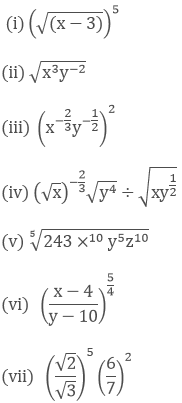Solution: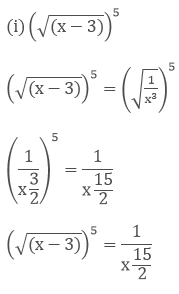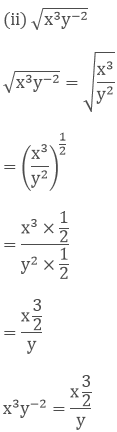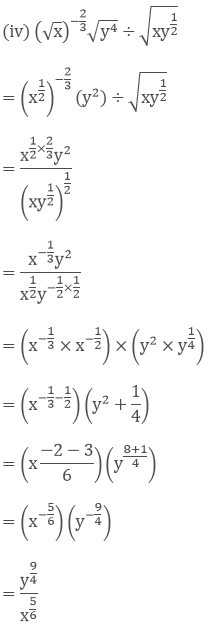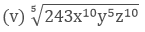= (243x10y5z10)1/5

= (243)1/5x10/5y5/5z10/5

= (35)1/5x2yz2

= 3x2yz2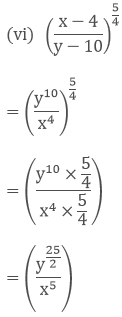Question: 2

Simplify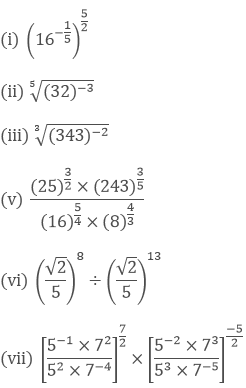Solution:= (4-1)

= 1/4= [(25)−3]1/5

= (2−15)1/5

= 2−3

= 1/23 = 1/8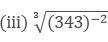= [(343)−2]1/3

= (343)−2×1/3

= (73)−2/3

= (7−2)

=(1/72)

= (1/49)

(iv) (0.001)1/3

= (1/1000)1/3

= (1/103)1/3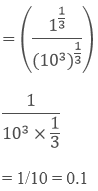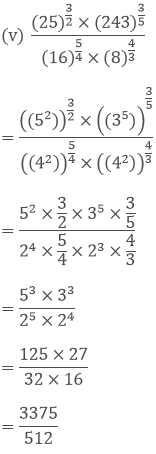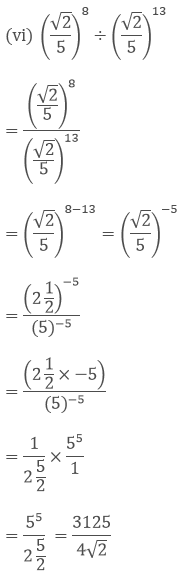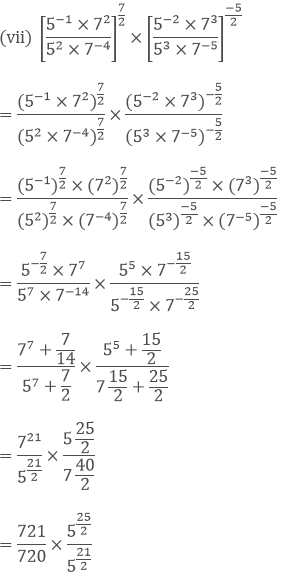= 721-20 × 525/2 - 21/2

= 71 × 54/2

= 71 × 52

= 7 × 25

= 175

Question: 3

Prove that(ii) 93/2 − 3 × 50 − (1/81)−1/2Solution: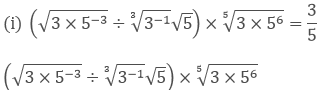= ((3 × 5−3)1/2 ÷ (3−1)1/3(5)1/2) × (3 × 56)1/6

= ((3)1/2(5−3)1/2 ÷ (3−1)1/3(5)1/2) × (3 × 56)1/6

= ((3)1/2(5)−3/2 ÷ (3)−1/3(5)1/2) × ((3)1/6 × (5)6/6)

= ((3)1/2 − (−1/3) × (5)−3/2−1/2) × ((3)1/6 × (5))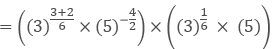= ((3)5/6 × (5)−2) × ((3)1/6 × (5))

= ((3)5/6+1/6 × (5)−2+1)

= ((3)6/6 × (5)−1)

= ((3)1 × (5)−1)

= ((3) × (5)−1)

= ((3) × (1/5))

= (3/5)

(ii) 93/2 − 3 × 50 − (1/81)−1/2

= (32)3/2 − 3 − (1/92)−1/2= 33 − 3 − (9)−2×−1/2

= 27 − 3 − 9

= 15= 24 − 3 × 23×2/3 + 4/3

= 16 − 3 × 22 + 4/3

= 16 − 3 × 4 + 4/3

= 16 − 12 + 4/3

= (12 + 4)/3

= 16/3= 2 × 1 × 5

= 10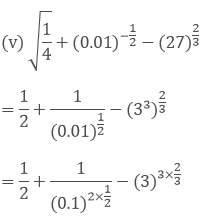= 1/2 + 1/(0.1)1 − (3)2

= 1/2 +1/(0.1) −9

= 1/2 + 10 − 9

= 1/2 + 1

= 3/2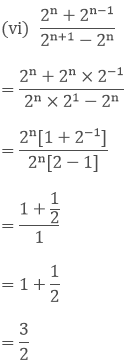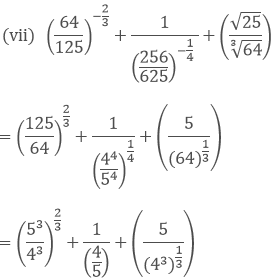= (5/4)2 + 5/4 + 5/4

= 25/16 + 10/4

= 25/16 + 40/16

= (26 + 40)/16

= 65/16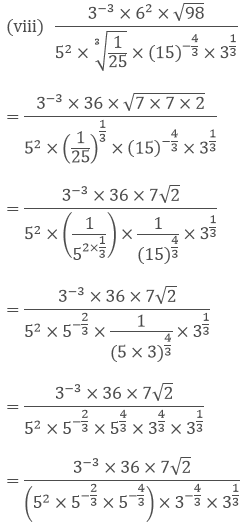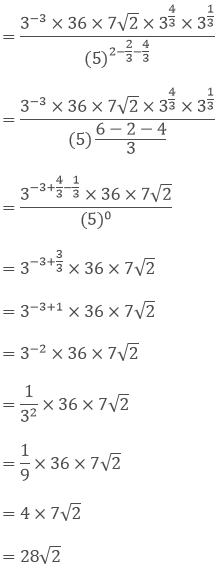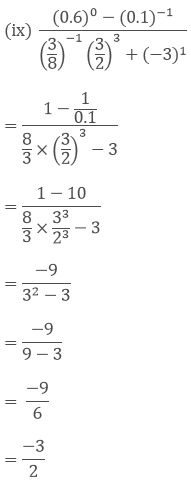Question: 4

Show that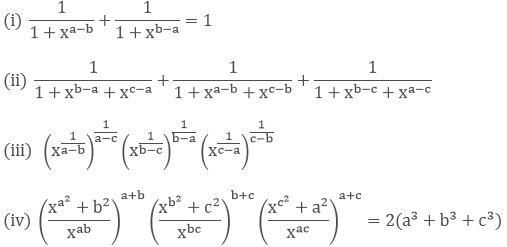(v) (xa−b)a + b (xb−c)b + c(xc − a)c + a = 1Solution: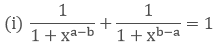Left hand side (LHS) = Right hand side (RHS)

Considering LHS,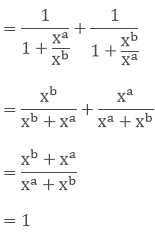Therefore, LHS = RHS

Hence proved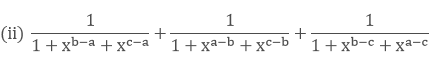Left hand side (LHS) = Right hand side (RHS)

Considering LHS,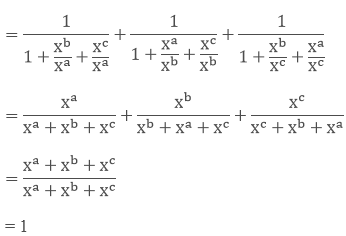Therefore, LHS = RHS

Hence proved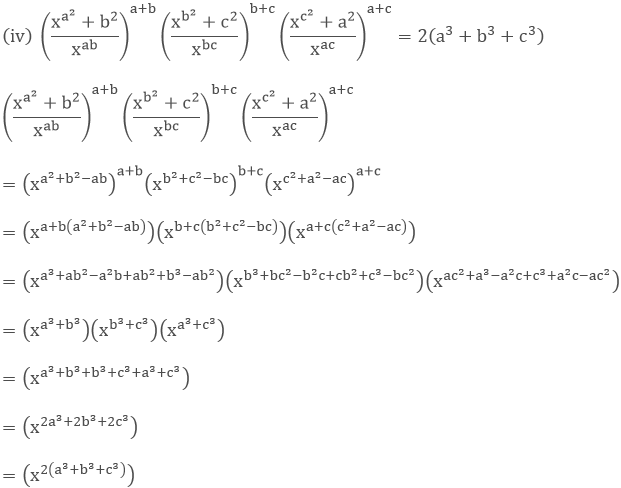(v) (xa−b)a + b (xb−c)b + c(xc − a)c + a = 1

(xa−b)a+b(xb−c)b+c(xc−a)c+a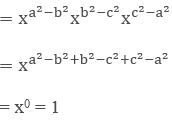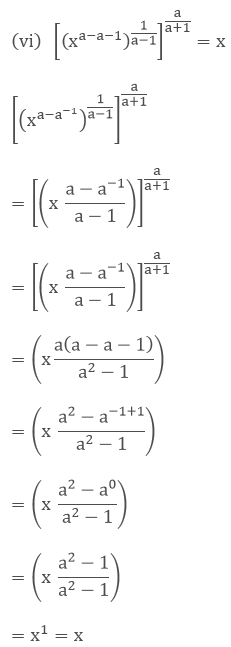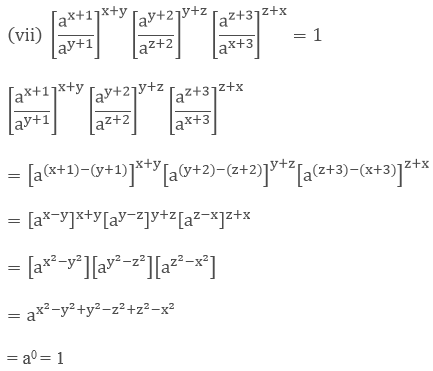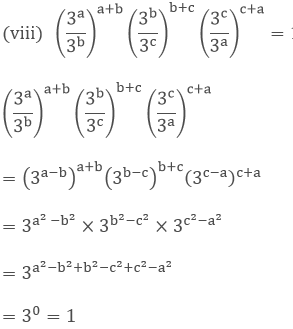Question: 5

If 2x = 3y = 12z, show that 1/z = 1/y + 2/x

Solution:

2x = 3y = (2 × 3 × 2)z

2x = 3y = (22 × 3)z

2x = 3y = (22z × 3z)

2x = 3y = 12z = k

2 = k1/x

3 = k1/y

12 = k1/z

12 = 2 × 3 × 2

12 = k1/z = k1/y × k1/x × k1/x

k1/z = k2/x + 1/y

1/z = 1/y + 2/x

Question: 6

If 2x = 3y = 6−z, show that 1/x + 1/y + 1/z = 0

Solution:

2x = 3y = 6−z

2x = k

2 = k1/x

3y = k

3 = k1/y

6−z = k

k = 1/6z

6 = k−1/z

2 × 3 = 6

k1/x × k1/y = k−1/z

1/x + 1/y = −1/z [by equating exponents]

1/x + 1/y + 1/z = 0

Question: 7

If ax = by = cz and b2 = ac, then show thatSolution:

Let ax = by = cz = k

a = k1/x, b = k1/y, c = k1/z

Now,

b2 = ac

(k1/y)2 = k1/x × k1/z

k2/y = k1/x + 1/z

2/y = 1/x + 1/zQuestion: 8

If 3x = 5y = (75)z, Show that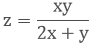Solution:

3x = k

3 = k1/x

5y = k

5 = k1/y

75z = k

75 = k1/z

31 × 52 = 751

k1/x × k2/y = k1/z

1/x + 2/y = 1/z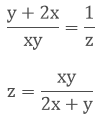Question: 9

If (27)x = 9/3x, find x

Solution:

We have,

(27)x = 9/3x

(33)x = 9/3x

33x = 9/3x

33x = 32/3x

33x = 32−x

3x = 2 − x [On equating exponents]

3x + x = 2

4x = 2

x = 2/4

x = 1/2

Here the value of x is ½

Question: 10

Find the values of x in each of the following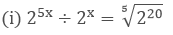(ii) (23)4 = (22)x

(iii) (3/5)x(5/3)2x = 125/27

(iv) 5x−2 × 32x−3 = 135

(v) 2x−7 × 5x−4 = 1250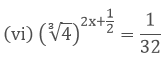(vii) 52x+3 = 1Solution:

We have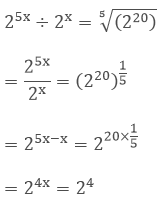= 4x = 4 [On equating exponent]

x = 1

Hence the value of x is 1

(ii) (23)4 = (22)x

We have

(23)4 = (22)x

= 23×4 = 22×x

12 = 2x

2x = 12 [On equating exponents]

x = 6

Hence the value of x is 6

(iii) (3/5)x(5/3)2x = 125/27

We have

(3/5)x(5/3)2x = 125/27⇒ 52x−x/32x−x  = 53/33

⇒ 5x/3x = 53/33

⇒ (5/3)x = (5/3)3

x = 3 [on equating exponents]

Hence the value of x is 3

(iv) 5x−2 × 32x−3 = 135

We have,

5x−2 × 32x−3 = 135

⇒ 5x−2 × 32x−3 = 5 × 27

⇒ 5x−2 × 32x−3 = 51 × 33

⇒ x − 2 = 1, 2x − 3 = 3 [On equating exponents]

⇒ x = 2 + 1, 2x = 3 + 3

⇒ x = 3, 2x = 6

⇒ x = 3

Hence the value of x is 3

(v) 2x−7 × 5x−4 = 1250

We have

2x−7 × 5x−4 = 1250

⇒ 2x−7 × 5x−4 = 2 × 625

⇒ 2x−7 × 5x−4 = 2 × 54

⇒ x − 7 = 1

⇒ x = 8, x − 4 = 4

⇒ x = 8

Hence the value of x is 8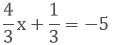4x + 1 = -15

4x = -15 - 1

4x = -16

x = (-16)/4

x = - 4

Hence the value of x is 4

(vii) 52x+3 = 1

52x+3 = 1 × 50

2x + 3 = 0 [By equating exponents]

2x = −3

x = −3/2

Hence the value of x is −3/2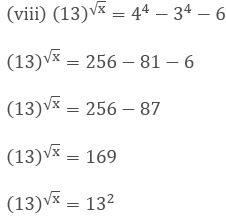√x = 2 [By equating exponents]

(√x)2 = (2)2

x = 4

Hence the value of x is 4x + 1 = - 6

x = - 6 - 1

x = -7

Hence the value of x is 7

Question: 11

If x = 21/3 + 22/3, show that x3 − 6x = 6

Solution:

x3 − 6x = 6

x = 21/3 + 22/3

Putting cube on both the sides, we get

x3  = (21/3 + 22/3)3

As we know, (a + b)3 = a3 + b3 + 3ab(a + b)

x3 = (21/3)3 + (22/3)3 + 3(21/3)(22/3)(21/3 + 22/3)

x3 = (21/3)3 + (22/3)3 + 3(21/3+2/3)(x)

x3 = (21/3)3 + (22/3)3 + 3(2)(x)

x3 = 6 + 6x

x3 - 6x = 6

Hence proved

Question: 12

Determine (8x)x, if 9x+2 = 240 + 9x.

Solution:

9x+2 = 240 + 9x

9x .92 = 240 + 9x

Let 9x be y

81y = 240 + y

81y - y = 240

80y = 240

y = 3

Since, y = 3

Then,

9x = 3

32x = 3

Therefore, x = ½

(8x)x = (8 × 1/2)1/2

= (4)1/2

= 2

Therefore (8x)x = 2

Question: 13

If 3x+1 = 9x-2, find the value of 21+x

Solution:

3x+1 = 9x-2

3x+1 = 32x-4

x + 1 = 2x - 4

x = 5

Therefore the value of 21+x = 21+5 = 26 = 64

Question: 14

If 34x = (81)-1 and (10)1/y = 0.0001, find the value of 2-x+4y.

Solution:

34x = (81)-1 and (10)1/y = 0.0001

34x = (3)-4

x = -1

And, (10)1/y = 0.0001

(10)1/y = (10)−4

1/y = -4

y = 1/−4

To find the value of 2-x+4y, we need to substitute the value of x and y

2-x+4y = 21+4(1/−4) = 21-1 = 20 = 1

Question: 15

If 53x = 125 and 10y = 0.001. Find x and y.

Solution:

53x = 125 and 10y = 0.001

53x = 53

x = 1

Now,

10y = 0.001

10y = 10-3

y = -3

Therefore, the value of x = 1 and the value of y = – 3

Question: 16

Solve the following equations

(i) 3x+1 = 27 × 34(iii) 3x−1 × 52y−3 = 225

(iv) 8x+1 = 16y+2 and (1/2)3+x = (1/4)3y

Solution:

(i) 3x+1 = 27 × 34

3x+1 = 33 × 34

3x+1 = 33+4

x + 1 = 3 + 4 [By equating exponents]

x + 1 = 7

x = 7 − 1

x = 64x = 3 (By equating exponents)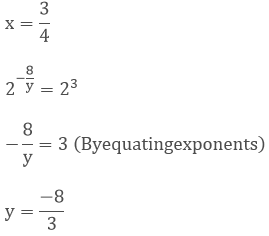(iii) 3x−1 × 52y−3 = 225

3x−1 × 52y−3 = 32 × 52

x − 1 = 2 [By equating exponents]

x = 3

3x−1 × 52y−3 = 32 × 52

2y − 3 = 2 [By equating exponents]

2y = 5

y = 5/2

(iv) 8x+1 = 16y+2 and (1/2)3+x = (1/4)3y

(23)x+1 and (2−1)3+x = (2−2)3y

3x + 3 = 4y + 8 and − 3 − x = −6y

3x + 3 = 4y + 8 and 3 + x = 6y

3x + 3 = 4y + 8 and y = (3+x)/6

3x + 3 = 4y + 8... eq1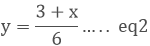Substitute eq2 in eq1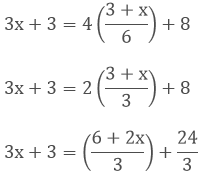3(3x + 3) = 6 + 2x + 24

9x + 9 = 30 + 2x

7x = 21

x = 21/7

x = 3

Putting value of x in eq2y = 1

(v) 4x−1 × (0.5)3−2x = (1/8)x

22x−2 × (5/10)3−2x = (1/23)x

22x−2 × (1/2)3−2x = 2−3x

22x−2 × 2−3+2x = 2−3x

2x − 2 − 3 + 2x = −3x [By equating exponents]

4x + 3x = 5

7x = 5x = 5/7(a/b)1/2 = (a/b)−(1−2x)1/2 = −1 + 2x [By equating exponents]

1/2 + 1 = 2x

2x = 3/2

x = ¾

Question: 17

If a and b are distinct positive primes such that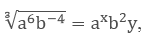, find x and y

Solution: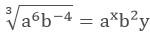(a6b−4)1/3 = axb2y

a6/3b−4/3 = axb2y

a2b−4/3 = axb2y

x = 2, 2y = −4/3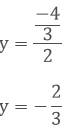Question: 18

If a and b are different positive primes such that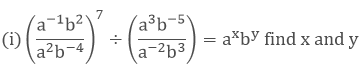(ii) (a + b)−1(a−1 + b−1) = axby, find x and y

Solution:(a−1−2b2+4)7 ÷ (a3+2b−5−3) = axby

(a−3b6)7 ÷ (a5b−8) = axby

(a−21b42) ÷ (a5b−8) = axby

(a−21−5b42+8) = axby

(a−26b50) = axby

x = −26, y = 50

(ii) (a + b)−1(a−1 + b−1) = axby, find x and y

(a + b)−1(a−1 + b−1)= 1/ab

= (ab)−1 = a−1b−1

By equating exponents

x = −1, y = −1

Therefore x + y + 2 = −1 − 1 + 2 = 0

Question: 19

If 2x × 3y × 5z = 2160, find x, y and z. Hence compute the value of 3x × 2−y × 5−z

Solution:

2x × 3y × 5z = 2160

2x × 3y × 5z = 24 × 33 × 51

x = 4, y = 3, z = 1

3x × 2−y × 5−z = 34 × 2−3 × 5−1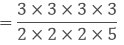= 81/40

Question: 20

If 1176 = 2a × 3b × 7c, find the values of a, b and c.

Solution:

Hence compute the value of 2a × 3b × 7−c as a fraction

1176 = 2a × 3b × 7c

23 × 31 × 72 = 2a × 3b × 7c

a = 3, b = 1, c = 2

We have to find the value of 2a × 3b × 7−c

2a × 3b × 7−c = 23 × 31 × 7−2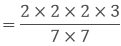= 24/49

Question: 21

Simplify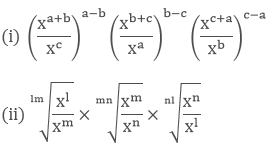Solution: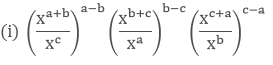(xa+b−c)a−b(xb+c−a)b−c(xc+a−b)c−a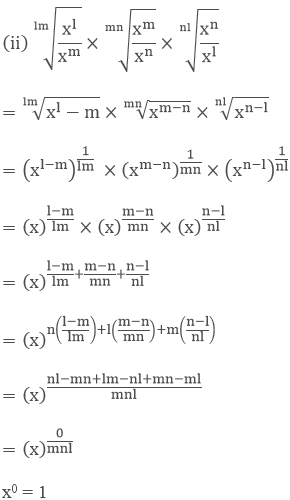Question: 22

Show that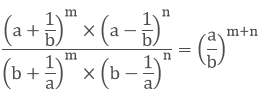Solution:Hence, LHS = RHS

Question: 23

(i) If a = xm+nyl, b = xn+lym and c = xl+myn, prove that am−nbn−lcl−m = 1

(ii) If x = am+n, y = an+l and z = al+m, prove that xmynzl = xnylzm

Solution:

(i) If a = xm+nyl, b = xn+lym and c = xl+myn, prove that am−nbn−lcl−m = 1

(xm+nyl)m−n(xn+lym)n−l(xl+myn)l−m

= (x(m+n)(m−n)yl(m−n))(x(n+l)(n−l)ym(n−l))(x(l+m)(l−m)yn(l−m))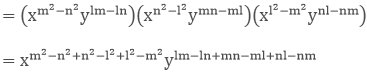= x0y0 = 1

(ii) If x = am+n, y = an+l and z = al+m, prove that xmynzl = xnylzm

LHS = xmynzl

(am+n)m(an+l)n(al+m)l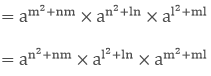=a(m+n)na(n+l)la(l+m)m

= xnylzm
```### Course Features

• 728 Video Lectures
• Revision Notes
• Previous Year Papers
• Mind Map
• Study Planner
• NCERT Solutions
• Discussion Forum
• Test paper with Video Solution